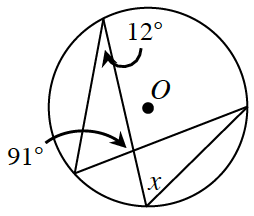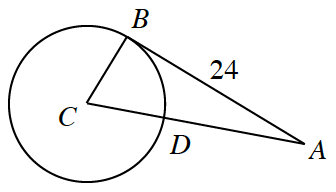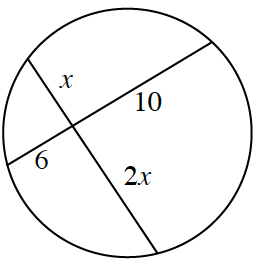### Home > INT2 > Chapter 12 > Lesson 12.1.4 > Problem12-50

12-50.This problem is a checkpoint for solving problems involving circles, arcs, sectors, chords, and tangents.

It will be referred to as Checkpoint 12.

1. In the figure at right, $O$ is the center of the circle. Calculate the value of $x$ and justify your answer.1. $C$ is the center of the circle and $\overline{AB}$ is tangent to the circle at point $B$. If $AD = 18$, calculate the area of the circle.1. Determine the value of $x$.Check your answers by referring to the Checkpoint 12 materials located at the back of your book.

Ideally, at this point you are comfortable working with these types of problems and can solve them correctly. If you feel that you need more confidence when solving these types of problems, then review the Checkpoint 12 materials and try the practice problems provided. From this point on, you will be expected to do problems like these correctly and with confidence.

Answers and extra practice are located in the back of your printed textbook or in the Reference Tab of your eBook. If you have an eBook for Int2, login and then click the following link: Checkpoint 12: Circles, Arcs, Sectors, Chords, and Tangents